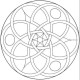## The distance formula and the Pythagorean theoremThoughts

For some reason I had, in the past, never understood where the terms in the distance formula came from. They always seemed like a bunch of intimidating terms that didn’t make much sense as a whole. Probably because the formula reads:

$latex d = \sqrt{ (x_2 – x_1)^2 + (y_2 – y_1)^2 }$

What the heck does all that gibberish mean? It’s actually not too difficult if you know of the idea that $latex a^2 + b^2 = c^2$ on a right triangle.So in this image we want to find the distance between the lower-left and upper-right red dots. If you remember the Pythagorean Theorem, when you have a right triangle, $latex a^2 + b^2 = c^2$. The two blue lines represent sides $latex a$ and $latex b$, and the pink line is the hypotenuse ($latex c$).

So if we want to find…

View original post 206 more words

This entry was posted in Uncategorized. Bookmark the permalink.